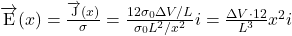A cylindrical glass rod is heated with a torch until it conducts enough current to cause a light bulb to glow. The rod has a length, a diame

Question

A cylindrical glass rod is heated with a torch until it conducts enough current to cause a light bulb to glow. The rod has a length, a diameter, and its ends, plated with material of infinite conductivity, are connected to the rest of the circuit. When red hot, the rod’s conductivity varies with position measured from the center of the rod as, with go = 4 x 10-2 (22 m).
1) What is the resistance of the glass rod?
2) When a voltage AV is applied between the two ends, what is the current density j?
3) What is the steady-state magnitude of the electric field E (1)?

in progress 0
5 months 2021-08-10T20:42:09+00:00 1 Answers 0 views 0

1) The resistance of the glass rod is 2122.1 Ω

2) The current density is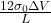3) the steady-state magnitude of the electric field E is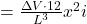.

Explanation:

Here we note that

the conductivity varies with position x measured from the center of the rod as

σ(x) = σ₀L²/x² with σ₀ = 4 × 10⁻² (Ω·m⁻¹)

the resistance of a small length x is given by

dR =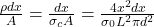Where:

ρ = Resistivity

From the center, we integrate over the entire length of the  cable as follows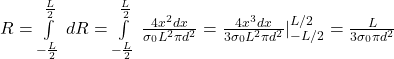Which gives

R =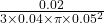= 2122.066 Ω

2) The current density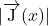=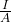Where:

I = Current =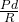and pd = ΔV

A = Area = πd²/4

Therefore current desity =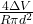Substituting  =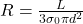Then we have

Current density =3) The steady-state magnitude of the electric field is given by

Magnitude of the Electric field